# QOTD-If a mirror is placed on the line XY, then which of the following answer figure is the right image of the given figure?

2019-07-06 | Team PendulumEdu

If a mirror is placed on the line XY, then which of the following answer figure is the right image of the given figure: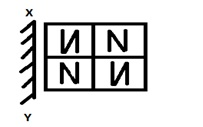A.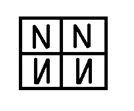B.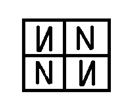D.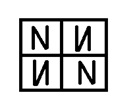D.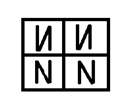Solution

When mirror is placed on the line XY then answer figure will look like: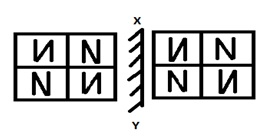Hence, (b) is the correct answer.

To answer such type of question one needs to visualize the image as it would look in front of the mirror. One should not get confused with the image formation in water.

Mirror image questions are asked in logical reasoning section of various competitive exams like SSC CGL, SSC CHSL, SSC MTS, SSC GD Constable, SSC JE, RRB JE, RRB NTPC, etc. Try free mock tests at PendulumEdu to practice and learn new techniques to solve the questions in minimum time.0